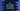# C++ find the sum of digits of a number until a single digit is obtained## C++ program to find the sum of digits of a number until a single digit is obtained:

In this post, we will learn how to find the sum of digits of a number until a single digit is obtained in C++. For example, if the number is 12345, the sum of all digits is 1+2+3+4+5=15. But, 15 is a two digit number. So, it will add the digits of this number 1+5 = 6, which is a one digit value.

So, the program will keep adding the digits, until a single digit value is obtained. If the input is 12345, it will print 6.

### Finding the sum recursively:

we can find the sum recursively. We will write one function to find the sum of all digits of a number. It will find the sum of the digits and if it is less than 10, it will return that value. Else, it will call itself to find the final result recursively.

Below is the complete C++ program to solve it:

``````#include <iostream>
using namespace std;

int findSum(int num){
int sum = 0;

while(num > 0){
sum += num % 10;
num = num/10;
}

return sum > 9 ? findSum(sum) : sum;
}

int main() {
cout<<findSum(12345);
return 0;
}``````

Here,

• findSum is the method to find the sum until a single digit value is found.
• This method takes one number as input. sum variable is used to store the sum of digits in the number.
• The while loop finds the sum of all digits of num. It runs until the value of num is greater than 0.
• On each iteration, the while loop adds the rightmost digit of num to sum. num % 10 gives the rightmost digit. Then it removes the rightmost digit from num. num = num/10 does that.
• Once the while loop ends, the sum will hold the sum of all digits of the number num. If it is a single digit value, it is returned. If it is more than 9 or if it is not single digit, it calls findSum to get the result recursively.

If you run this program, it will print the below output:

``6``

### Method 2: By checking if the number is divisible by 9:

The sum of all digits of a number until the value reached one digit is 9 if the number is divisible by 9. Else, it is the remainder if we divide the number by 9.

For the above example number 9, if we divide it by 9, the remaiinder is 12345%9 = 6, which is the sum of all digits of this number until we reached one digit.

So, we can write this program only in one line:

``````#include <iostream>
using namespace std;

int findSum(int num)
{
int remainder = num % 9;

return remainder == 0 ? 9 : remainder;
}

int main()
{
cout << findSum(12345) << endl;
return 0;
}``````

We are changing only the findSum method. It first calculates the remainder value, i.e. the remainder if we divide the number by 9 and then compares it with 0. If it is 0, it returns 9, else it returns the remainder.